# Applications of Taylor Series

 Final exam: Wednesday, March 22, 7-10pm in PCYNH 109. Bring ID! Last Quiz 4: Today (last one) Today: 11.12 Applications of Taylor Polynomials Next; Differential Equations

This section is about an example in the theory of relativity. Let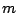be the (relativistic) mass of an object and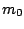be the mass at rest (rest mass) of the object. Let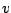be the velocity of the object relative to the observer, and let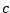be the speed of light. These three quantities are related as follows: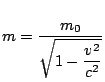(relativistic) mass

The total energy of the object is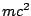: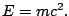In relativity we define the kinetic energy to be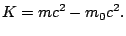(6.4)

What? Isn't the kinetic energy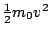?

Notice that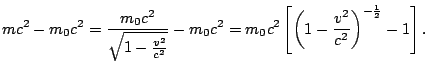Let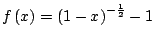Let's compute the Taylor series of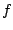. We have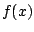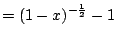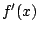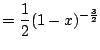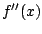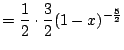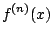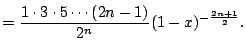Thus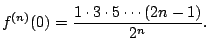Hence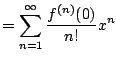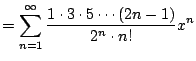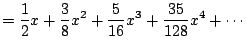We now use this to analyze the kinetic energy ():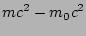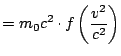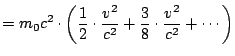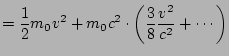And we can ignore the higher order terms if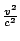is small. But how small is small'' enough, given thatappears in an infinite sum?

Subsections
William Stein 2006-03-15